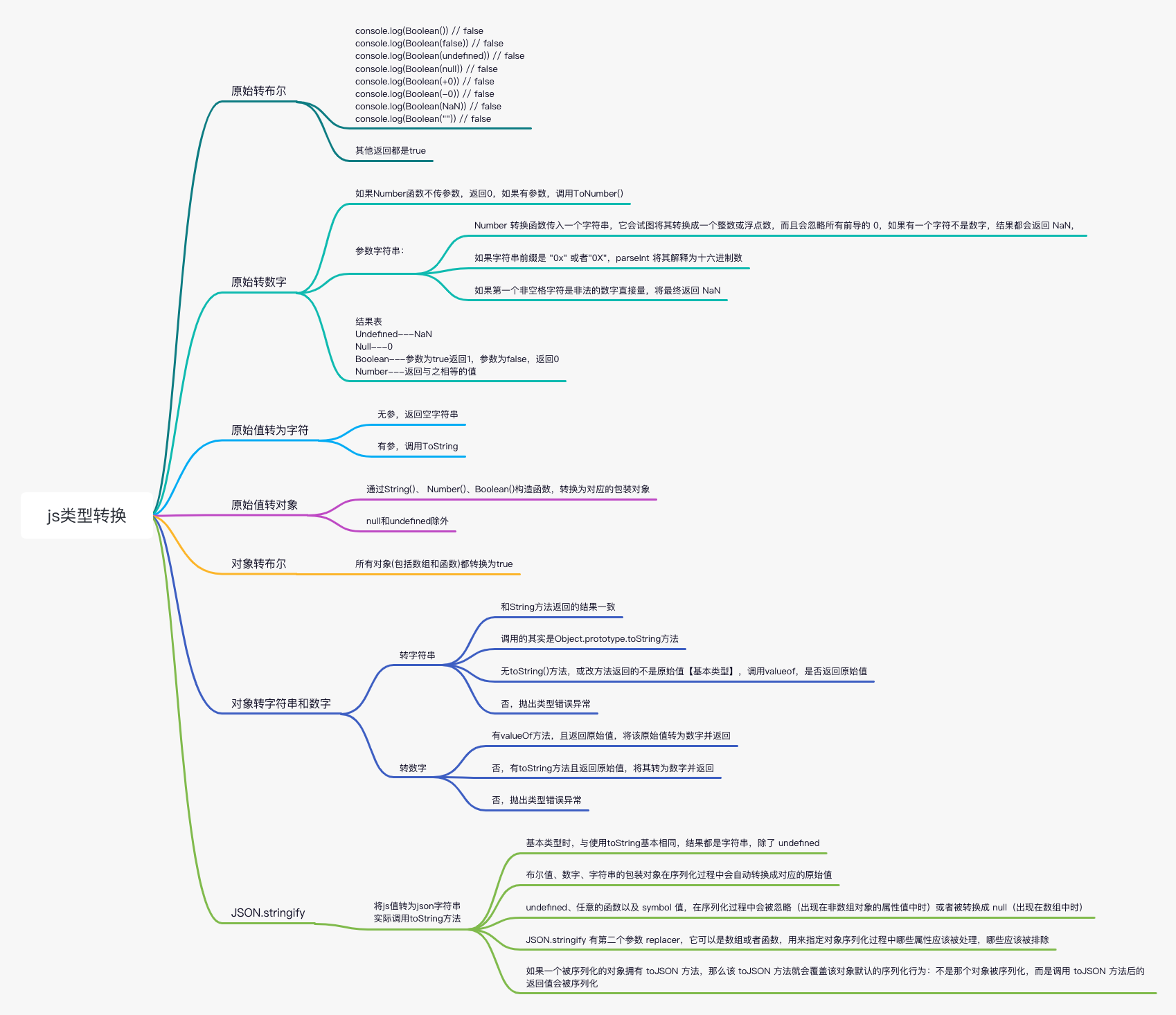# JavaScript的类型转换

9/23/2021 Javascript

## # 一元操作符 +

``````console.log(+'1') // 1
console.log(+[]) // 0
console.log(+['1']) // 1
console.log(+['1', '2']) // NaN
console.log(+{}) // NaN
``````
1
2
3
4
5

## # 二元操作符 +

1. left = ToPrimitive(A)
2. right = ToPrimitive(B)
3. 如果 left 或 right 是字符串，那么返回 ToString(left)和 ToString(right)的拼接结果
4. 否则返回 ToNumber(left)+ToNumber(right)的值

## # ==相等

"=="用于比较两个值相等，当要比较的两个值类型不一样的时候，会发生类型的转换。

1. 如果 x 和 y 是同一类型：
• undefined === undefined,返回 true
• null === null,返回 true
• NaN === NaN,返回 false
• +0 == -0，返回 false
• -0 == +0，返回 false
• x 和 y 是字符串，完全相等返回 true，否则返回 false
• x 和 y 是布尔值，x 和 y 都是 true 返回 false，返回 true，否则返回 false
• x 和 y 指向同一个对象，返回 true，否则返回 false
1. null == undefined,返回 true
2. undefined == null,返回 false
3. x 是数字，y 是字符串，判断 x == ToNumber(y)
4. x 是字符串，x 是数字，判断 ToNumber(x) == y
5. x 是布尔值，判断 ToNumber(x) == y
6. y 是布尔值，判断 x == ToNumber(y)
7. x 是字符串或者数字，y 是对象，判断 x == ToPrimitive(y)
8. x 是对象，y 是字符串或数字，判断 ToPrimitive(x) == y

## # 各种数据类型之间的转换